# 简单遗传算法MATLAB实现

+关注继续查看

1. 遗传算法实现过程

clip_image002

clip_image004

clip_image006

1.1 编码与解码

clip_image007

1.2 初始化种群

1.3 选择操作

clip_image020

Selection Algorithm
var pop, pop_new;/pop为前代种群，pop_new为下一代种群/
var fitness_value, fitness_table;/fitness_value为种群的适应度，fitness_table为种群累积适应度/
for i=1:pop_size

r = rand*fitness_table(pop_size);/*随机生成一个随机数，在0和总适应度之间，因为fitness_table(pop_size)为最后一个个体的累积适应度，即为总适应度*/
first = 1;
last = pop_size;
mid = round((last+first)/2);
idx = -1;
/*下面按照排中法选择个体*/
while (first <= last) && (idx == -1)
if r > fitness_table(mid)
first = mid;
elseif r < fitness_table(mid)
last = mid;
else
idx = mid;
break;
end if
mid = round((last+first)/2);
if (last - first) == 1
idx = last;
break;
end if
end while

for j=1:chromo_size
pop_new(i,j)=pop(idx,j);
end for

end for
/是否精英选择/
if elitism

    p = pop_size-1;

else

    p = pop_size;

end if
for i=1:p

   for j=1:chromo_size
pop(i,j) = pop_new(i,j);/*若是精英选择，则只将pop_new前pop_size-1个个体赋给pop，最后一个为前代最优个体保留*/
end for

end for
1.3 交叉操作

clip_image001

Crossover algorithm
for i=1:2:pop_size

        if(rand < cross_rate)/*cross_rate为交叉概率*/
cross_pos = round(rand * chromo_size);/*交叉位置*/
if or (cross_pos == 0, cross_pos == 1)
continue;/*若交叉位置为0或1，则不进行交叉*/
end if
for j=cross_pos:chromo_size
pop(i,j)<->pop(i+1,j);/*交换*/
end for
end if

end for
1.4 变异操作

clip_image001

Mutation algorithm
for i=1:pop_size

        if rand < mutate_rate/*mutate_rate为变异概率*/
mutate_pos = round(rand*chromo_size);/*变异位置*/
if mutate_pos == 0
continue;/*若变异位置为0，则不进行变异*/
end if
pop(i,mutate_pos) = 1 - pop(i, mutate_pos);/*将变异位置上的数字至反*/
end if

end for
1.5 遗传算法流程

clip_image001

1.6 MATLAB程序实现

%初始化种群
%pop_size: 种群大小
%chromo_size: 染色体长度

function initilize(pop_size, chromo_size)
global pop;
for i=1:pop_size

for j=1:chromo_size
pop(i,j) = round(rand);
end

end
clear i;
clear j;

%计算种群个体适应度，对不同的优化目标，此处需要改写
%pop_size: 种群大小
%chromo_size: 染色体长度

function fitness(pop_size, chromo_size)
global fitness_value;
global pop;
global G;
for i=1:pop_size

fitness_value(i) = 0.;    

end

for i=1:pop_size

for j=1:chromo_size
if pop(i,j) == 1
fitness_value(i) = fitness_value(i)+2^(j-1);
end
end
fitness_value(i) = -1+fitness_value(i)*(3.-(-1.))/(2^chromo_size-1);
fitness_value(i) = -(fitness_value(i)-1).^2+4;

end

clear i;
clear j;

%对个体按适应度大小进行排序，并且保存最佳个体
%pop_size: 种群大小
%chromo_size: 染色体长度

function rank(pop_size, chromo_size)
global fitness_value;
global fitness_table;
global fitness_avg;
global best_fitness;
global best_individual;
global best_generation;
global pop;
global G;

for i=1:pop_size

fitness_table(i) = 0.;

end

min = 1;
temp = 1;
temp1(chromo_size)=0;
for i=1:pop_size

min = i;
for j = i+1:pop_size
if fitness_value(j)<fitness_value(min);
min = j;
end
end
if min~=i
temp = fitness_value(i);
fitness_value(i) = fitness_value(min);
fitness_value(min) = temp;
for k = 1:chromo_size
temp1(k) = pop(i,k);
pop(i,k) = pop(min,k);
pop(min,k) = temp1(k);
end
end


end

for i=1:pop_size

if i==1
fitness_table(i) = fitness_table(i) + fitness_value(i);
else
fitness_table(i) = fitness_table(i-1) + fitness_value(i);
end

end
fitness_table
fitness_avg(G) = fitness_table(pop_size)/pop_size;

if fitness_value(pop_size) > best_fitness

best_fitness = fitness_value(pop_size);
best_generation = G;
for j=1:chromo_size
best_individual(j) = pop(pop_size,j);
end

end

clear i;
clear j;
clear k;
clear min;
clear temp;
clear temp1;

%轮盘赌选择操作
%pop_size: 种群大小
%chromo_size: 染色体长度
%cross_rate: 是否精英选择

function selection(pop_size, chromo_size, elitism)
global pop;
global fitness_table;

for i=1:pop_size

r = rand * fitness_table(pop_size);
first = 1;
last = pop_size;
mid = round((last+first)/2);
idx = -1;
while (first <= last) && (idx == -1)
if r > fitness_table(mid)
first = mid;
elseif r < fitness_table(mid)
last = mid;
else
idx = mid;
break;
end
mid = round((last+first)/2);
if (last - first) == 1
idx = last;
break;
end

end

for j=1:chromo_size

    pop_new(i,j)=pop(idx,j);

end
end
if elitism

p = pop_size-1;

else

p = pop_size;

end
for i=1:p
for j=1:chromo_size

    pop(i,j) = pop_new(i,j);

end
end

clear i;
clear j;
clear pop_new;
clear first;
clear last;
clear idx;
clear mid;

%单点交叉操作
%pop_size: 种群大小
%chromo_size: 染色体长度
%cross_rate: 交叉概率

function crossover(pop_size, chromo_size, cross_rate)
global pop;
for i=1:2:pop_size

if(rand < cross_rate)
cross_pos = round(rand * chromo_size);
if or (cross_pos == 0, cross_pos == 1)
continue;
end
for j=cross_pos:chromo_size
temp = pop(i,j);
pop(i,j) = pop(i+1,j);
pop(i+1,j) = temp;
end
end

end

clear i;
clear j;
clear temp;
clear cross_pos;

%单点变异操作
%pop_size: 种群大小
%chromo_size: 染色体长度
%cross_rate: 变异概率
function mutation(pop_size, chromo_size, mutate_rate)
global pop;

for i=1:pop_size

if rand < mutate_rate
mutate_pos = round(rand*chromo_size);
if mutate_pos == 0
continue;
end
pop(i,mutate_pos) = 1 - pop(i, mutate_pos);
end

end

clear i;
clear mutate_pos;

%打印算法迭代过程
%generation_size: 迭代次数

function plotGA(generation_size)
global fitness_avg;
x = 1:1:generation_size;
y = fitness_avg;
plot(x,y)

%遗传算法主函数
%pop_size: 输入种群大小
%chromo_size: 输入染色体长度
%generation_size: 输入迭代次数
%cross_rate: 输入交叉概率
%cross_rate: 输入变异概率
%elitism: 输入是否精英选择
%m: 输出最佳个体
%n: 输出最佳适应度
%p: 输出最佳个体出现代
%q: 输出最佳个体自变量值

function [m,n,p,q] = GeneticAlgorithm(pop_size, chromo_size, generation_size, cross_rate, mutate_rate, elitism)

global G ; %当前代
global fitness_value;%当前代适应度矩阵
global best_fitness;%历代最佳适应值
global fitness_avg;%历代平均适应值矩阵
global best_individual;%历代最佳个体
global best_generation;%最佳个体出现代

fitness_avg = zeros(generation_size,1);

disp "hhee"

fitness_value(pop_size) = 0.;
best_fitness = 0.;
best_generation = 0;
initilize(pop_size, chromo_size);%初始化
for G=1:generation_size

fitness(pop_size, chromo_size);  %计算适应度
rank(pop_size, chromo_size);  %对个体按适应度大小进行排序
selection(pop_size, chromo_size, elitism);%选择操作
crossover(pop_size, chromo_size, cross_rate);%交叉操作
mutation(pop_size, chromo_size, mutate_rate);%变异操作

end
plotGA(generation_size);%打印算法迭代过程
m = best_individual;%获得最佳个体
n = best_fitness;%获得最佳适应度
p = best_generation;%获得最佳个体出现代

%获得最佳个体变量值，对不同的优化目标，此处需要改写
q = 0.;
for j=1:chromo_size

if best_individual(j) == 1
q = q+2^(j-1);
end 

end
q = -1+q*(3.-(-1.))/(2^chromo_size-1);

clear i;
clear j;

1. 案例研究

function run_ga()
elitism = true;%选择精英操作
pop_size = 20;%种群大小
chromo_size = 16;%染色体大小
generation_size = 200;%迭代次数
cross_rate = 0.6;%交叉概率
mutate_rate = 0.01;%变异概率

[m,n,p,q] = GeneticAlgorithm(pop_size, chromo_size, generation_size, cross_rate, mutate_rate,elitism);
disp "最优个体"
m
disp "最优适应度"
n
disp "最优个体对应自变量值"
q
disp "得到最优结果的代数"
p

clear;

"最优个体"

m =

1 1 1 1 1 1 1 1 1 1 1 1 1 1 1 0

"最优适应度"

n =

4.0000

"最优个体对应自变量值"

q =

1.0000

"得到最优结果的代数"

p =

74

clip_image002

1. 总结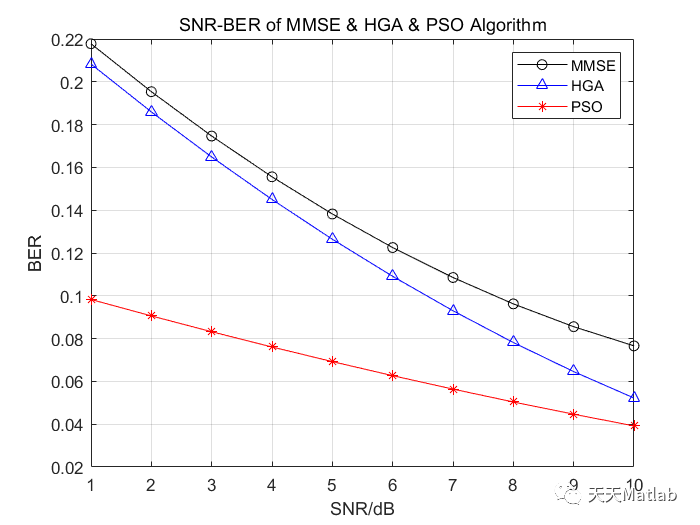【OFDM仿真】基于秩亏情况下遗传算法和粒子群算法优化MIMO-OFDM系统多用户检测附matlab代码
【OFDM仿真】基于秩亏情况下遗传算法和粒子群算法优化MIMO-OFDM系统多用户检测附matlab代码
0 0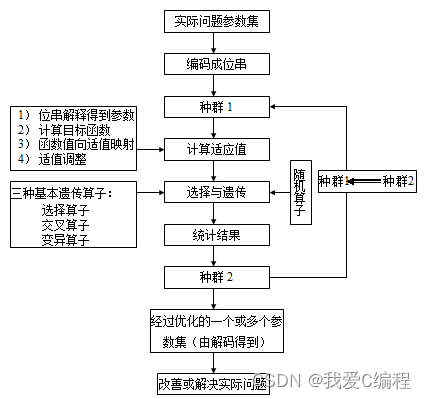m基于自适应遗传优化的IEEE-6建设费用和网络损耗费用最小化电网规划算法matlab仿真
m基于自适应遗传优化的IEEE-6建设费用和网络损耗费用最小化电网规划算法matlab仿真
0 0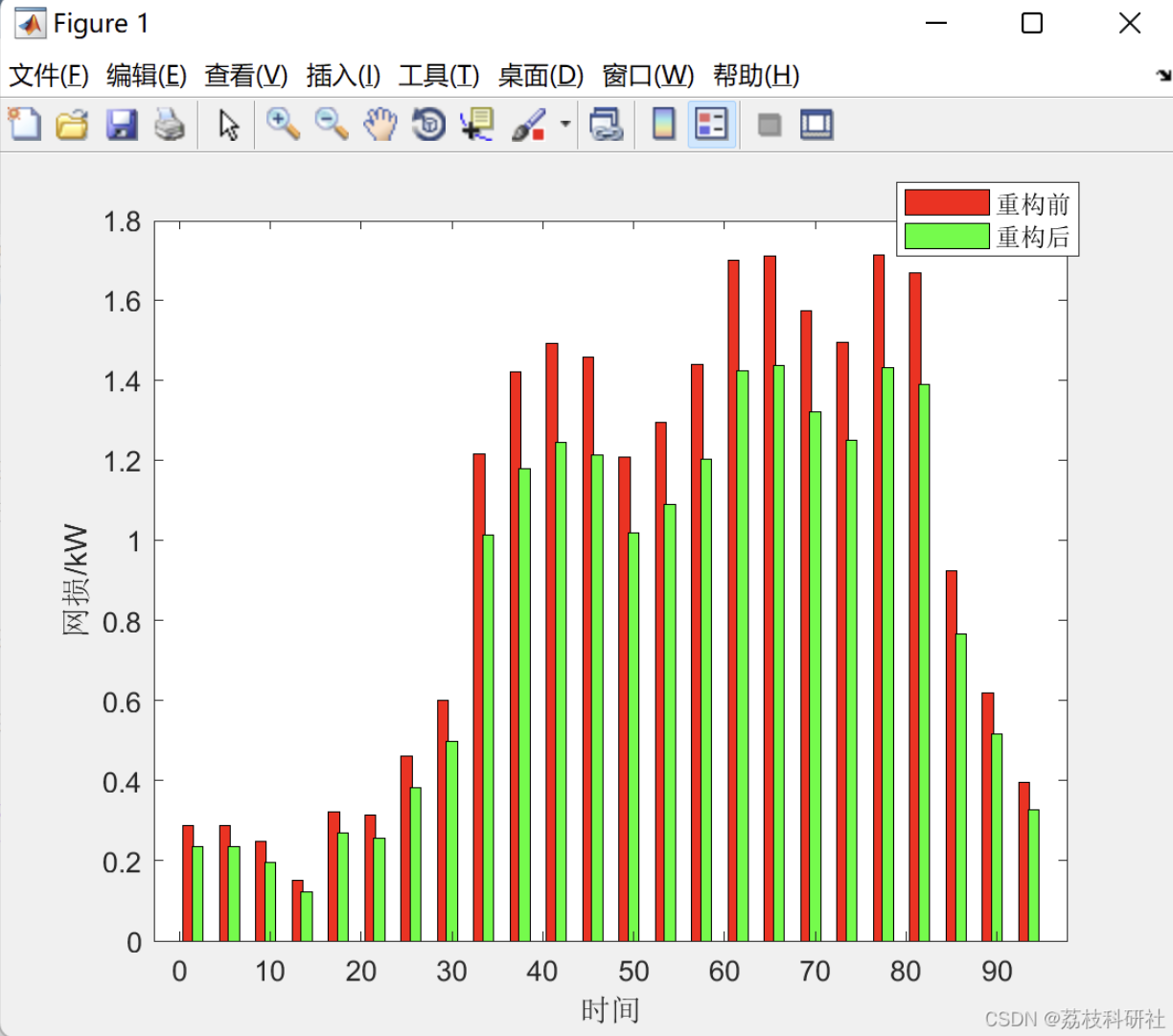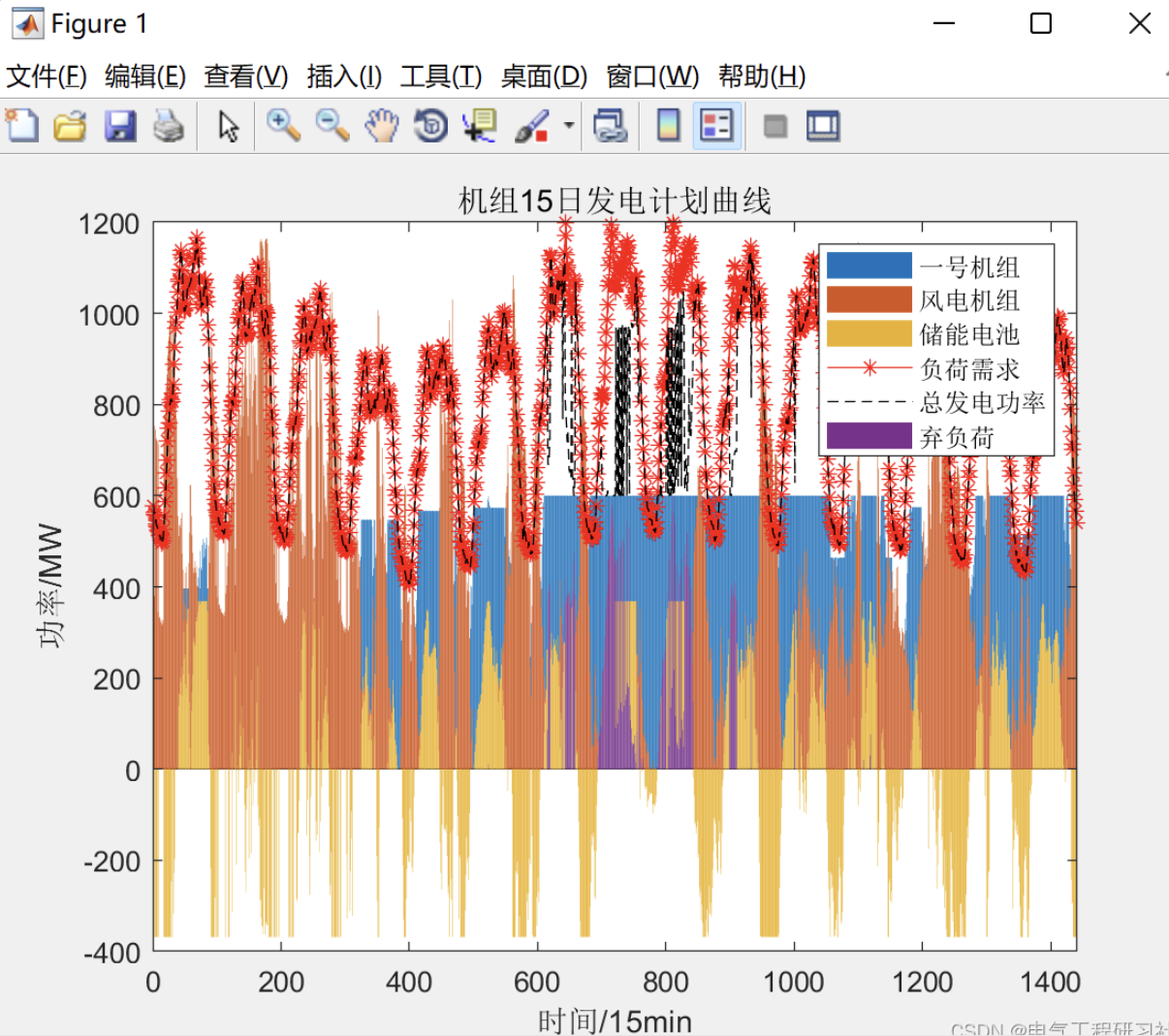0 0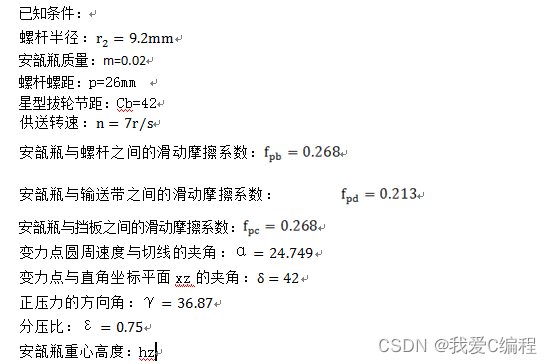m基于GA遗传算法的分件供送螺杆参数优化matlab仿真,优化参数包括螺杆总尺寸-最大圈数等
m基于GA遗传算法的分件供送螺杆参数优化matlab仿真,优化参数包括螺杆总尺寸-最大圈数等
0 0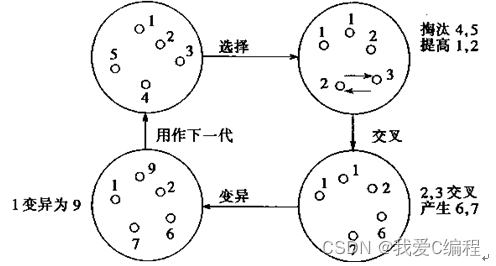m基于GA遗传算法的电动汽车有序充电控制策略matlab仿真
m基于GA遗传算法的电动汽车有序充电控制策略matlab仿真
0 0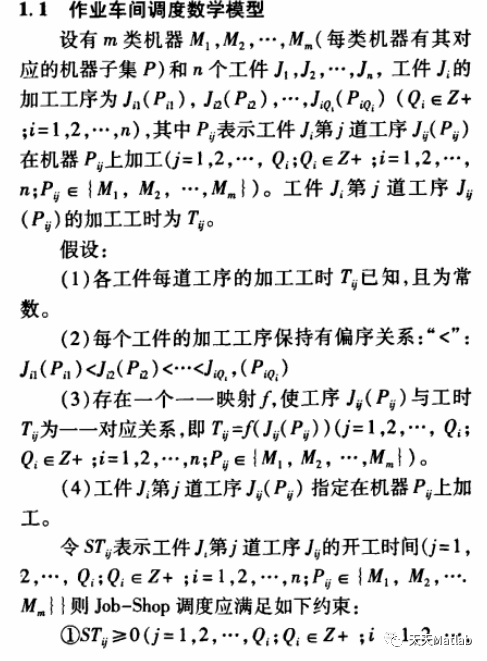【车间调度】基于候鸟和遗传算法求解柔性作业车间调度问题MBO-FJSP附matlab代码
【车间调度】基于候鸟和遗传算法求解柔性作业车间调度问题MBO-FJSP附matlab代码
0 0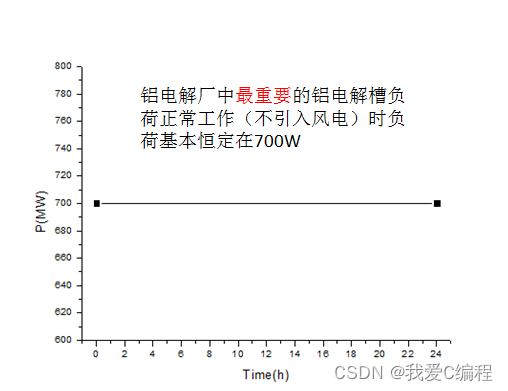m基于GA遗传算法的高载能负荷响应优化控制模型matlab仿真
m基于GA遗传算法的高载能负荷响应优化控制模型matlab仿真
0 0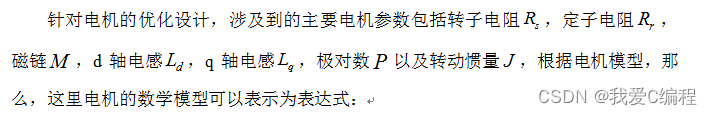m基于GA遗传算法的PMSM永磁同步电机参数最优计算matlab仿真
m基于GA遗传算法的PMSM永磁同步电机参数最优计算matlab仿真
0 0【车间调度】基于遗传算法求解柔性生产调度问题GA-FJSP附matlab代码
【车间调度】基于遗传算法求解柔性生产调度问题GA-FJSP附matlab代码
0 0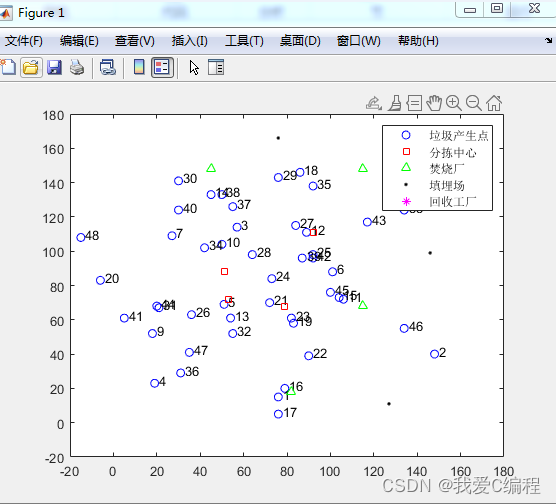m基于遗传算法的城市生活垃圾回收网络优化matlab仿真
m基于遗传算法的城市生活垃圾回收网络优化matlab仿真
0 0